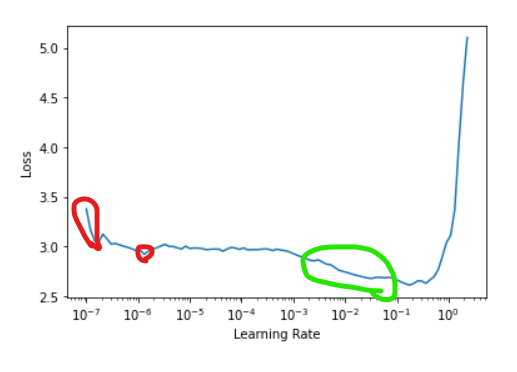# Discrepancy between x-axis of learning rate finder and suggested learning rates

Hello – it seems to me that there is an order-of-magnitude discrepancy between the x-axis of the learning rate finder plot and the two suggested best learning rates (the steepest point and the minimum point). Here’s an example from Chapter 5 (Lesson 6):

In the plot, the minimum is just below the tick for 10-1 (which is equal to 0.1). However, the function reports the learning rate that minimizes this function is 0.008, which is about an order of magnitude smaller than what is shown in the plot.

Does the x-axis of the learning rate finder plot need to be corrected so each value is an order of magnitude smaller than it currently appears? Or are the returned values incorrect by an order of magnitude? Or am I seeing something totally wrong here?

Thanks!

1 Like

Not quite. There should be a flag in `lr_find` which should show the recommendations, and what you will find is the recommendation is not actually the minimum, but the exact midpoint of that long line stemming from 1e-3 to 1e-1. We choose the center because this will generally be the steepest point on the plot. We don’t necessary want the fastest speed to where the loss stopped improving

1 Like

Thank you. I do have the `lr_find()` parameter `suggestions` set to `True` (the default). The recommendation/suggestion for the midpoint of the descending line should be stored in `lr_steep` by the following (in my first post above): `lr_min,lr_steep = learn.lr_find()`

This returns `lr_steep` = 0.0025. However, according to the x-axis of the learning rate finder plot (as shown in my first post above), the midpoint of this line is approx. 0.01, which is about an order of magnitude off from the numerical suggestion in `lr_steep`. This is what makes me think there is a discrepancy. Am I still missing something?

I would recommend whenever you have questions about a function or class or what some piece of code is doing, look more closely at the source code. To do this, you can use the `doc()` command (specific to fastai objects) or use the `??` magic command. So if we were to execute a code cell with `doc(learn.lr_find)` or `learn.lr_find??` then we would see the following source code:

``````def lr_find(self:Learner, start_lr=1e-7, end_lr=10, num_it=100, stop_div=True, show_plot=True, suggestions=True):
"Launch a mock training to find a good learning rate, return lr_min, lr_steep if `suggestions` is True"
n_epoch = num_it//len(self.dls.train) + 1
cb=LRFinder(start_lr=start_lr, end_lr=end_lr, num_it=num_it, stop_div=stop_div)
with self.no_logging(): self.fit(n_epoch, cbs=cb)
if show_plot: self.recorder.plot_lr_find()
if suggestions:
lrs,losses = tensor(self.recorder.lrs[num_it//10:-5]),tensor(self.recorder.losses[num_it//10:-5])
if len(losses) == 0: return
lr_min = lrs[losses.argmin()].item()
grads = (losses[1:]-losses[:-1]) / (lrs[1:].log()-lrs[:-1].log())
return SuggestedLRs(lr_min/10.,lr_steep)
``````

Of importance to your question is the last line. Indeed, the returned value is actually the minimum learning rate divided by 10.

Why would we want to do this? Theoretically, the best learning rate is one where the loss is decreasing the most quickly, because we would want the loss to decreased most quickly during training as well. Therefore, we want to select the steepest part of the curve for the learning rate. This is why `lr_steep` is returned. But in practice, sometimes you get curves like this:This is actually from a model I was just training. Do you see the red circled areas? These are actually the steepest regions of this curve. But this is clearly not what we want. We instead want that region where the loss is going down fairly rapidly before it shoots up again (circled in green). This region is often right before the loss hits a minimum and explodes (as you can see above). So this is why lr_min/10 is a fairly reasonable number to select a learning rate if `lr_steep` provides a value that is somewhat nonsensical.

Let me know if you have any questions!

4 Likes

That makes a lot of sense! Thanks so much for the explanation.